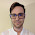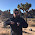Thursday, September 24, 2015

The unobservables

"Tight money leads to lower expected future NGDP growth. I don’t think that can be disputed."

No, Scott, it really can't be disputed ... because you define "tight" money by lower future expected NGDP growth.

Coupled with my post on Wicksell earlier this week, Sumner's post made me think a bit about what I'll call the unobservables: a collection of macroeconomic properties that cannot be directly measured. This list is not meant to be exhaustive, but here are some key ones :
• The natural rate of interest
• The natural rate of unemployment/NAIRU
• The "stance" of monetary policy
• Inflation expectations
• The velocity of money
What do these all have in common? They're all directly linked to an observable: inflation. Scott Sumner does mix it up a bit by keying in on NGDP growth (= inflation + RGDP growth). So the unobservables are all linked to a specific mechanism of (accelerating) inflation:
• Interest rates too low (price of money too low)
• Unemployment too low (wage-price spiral)
• Expansionary monetary policy (supply and demand for money)
• Agents expect inflation (rational expectations, adaptive expectations)
• Money supply increases (at constant velocity)
This leaves out a couple of additional mechanisms like supply shocks (which aren't monetary policy and thus considered more "real") and the neo-Fisherite view that high interest rates lead to inflation (via a specific expectations mechanism). But essentially we have a list of ideas people had about what causes inflation combined with an unobservable factor that determines how/when the mechanism works. Here's a generic way of putting this:
We get a fire when temperature is above the natural temperature of phlogiston.
How do we determine the natural temperature of phlogiston? We observe a fire.
That's how you get falling estimates of the natural rate of interest [pdf] or unemployment, or falling velocity. And that's how you get Scott Sumner calling monetary policy "tight" since the 2008 recession. I don't mean to say that is hasn't been "tight", just that "tight" is kind of a circular definition.

Of all of the mechanism/unobservable combinations above, only one rises a bit above the circular phlogiston example. That is inflation expectations -- purportedly measured by TIPS spreads (the difference between the interest rate on a regular treasury bond and its inflation-protected cousin). But the problem is that inflation expectations measured by TIPS spreads seem to be entirely backward looking (or see here) or at least strongly dependent on previous inflation when inflation isn't high (see e.g. here H/T Mark Thoma). The only unobservable that has at least a theoretical way to measure it turns out to get it wrong. So either inflation expectations don't have a strong effect on inflation or things like TIPS spreads don't measure inflation expectations correctly.

And really, when you're comparing your measure of inflation expectations to actual inflation to see how much of an impact inflation expectations had, you've basically started to measure the temperature of phlogiston.

Now don't get me wrong: I'm not against the idea of an unobservable! As a physicist, I've frequently used an unobservable: the wavefunction in quantum mechanics. However, you do not figure out what the wavefunction is by measuring an interference pattern and taking its square root. There is a very specific model for calculating the wavefunction (e.g. the Schrodinger equation).

And that's the issue with the macroeconomic unobservables. Most of the time there's no way to calculate them besides using the data you're trying to explain, and when you can calculate them, they turn out to be wrong (inflation expectations are backwards looking, money velocity isn't constant).

I like to think of the information transfer model as the analog of the Schrodinger equation for velocity. But really, it's the Schrodinger equation for all of these unobservables since it tells you what inflation and NGDP are going to be. "Tight" money is when interest rates are above the information equilibrium value, or when the economy is above the NGDP-M0 path (see here). The best measure of the natural rate of interest is the information equilibrium value. The best measure of the natural rate of unemployment can be calculated. Velocity is κP (though I like Cambridge k more). And κ is a measure of the relative information in a symbol of output (proportional to the log of output) to a symbol of money (proportional to the log of the amount of cash). Or (probably more accurately), the inverse of the ensemble average of all of the individual market relative growth rate factors a.

But inflation expectations -- those are bogus.

Footnotes:

 I left out total factor productivity because I'm focusing on monetary policy.

1.I'm not sure what you mean by calling velocity unobservable. Can you clarify that a bit for me?

1.The velocity is unobservable in similar fashion as the force of gravity on a falling object in a vacuum is unobservable. We can derive it from the observation of its acceleration and mass. But how do we know its mass? We might weigh it, but that is a way of measuring the force of gravity upon it.

Eddington paraphrased Newton's First Law of Motion something like this: "A body remains in a state of rest or of uniform motion, except inasmuch as it doesn't." ;) His point was that we infer the force from the acceleration.

We can derive the velocity of money from the equation, MV = PQ, but we do not observe it directly.

I wondered about Jason's inclusion of inflation expectations and money velocity, but he cleared that up for me when he said, "inflation expectations are backwards looking, money velocity isn't constant".

To which I, as a non-economist, reply, "That's a big so what, good buddy!" ;)

2.I suppose I get the 'we don't observe it directly' logic, but that doesn't really apply to velocity because, unlike the other properties, it is a definition. I can know for certain what the velocity of the monetary base is; I can't know for certain what expected inflation, NAIRU, or the natural rate of interest are. The later the are undefined because of insufficient theory whereas velocity is unexplained because of insufficient theory.

3.There is a way to calculate velocity (PY/M) and you're right that it is more of a definition nowadays. I was careful to couple velocity with the model that makes it useful: that it is constant in the short run.

If you take velocity to just be the definition, the model doesn't really have any theoretical content at all.

4.Wouldn't 'stable money demand function' be better than 'velocity' then?

5.Since most economists today seem to eschew the equation of exchange, that's probably the more common way to do it.

Interestingly, in the information transfer model velocity is actually a price of money (a detector of information flow), not a demand for money (a source of of information):

p ≡ dN/dM = k N/M

N ≡ PY

"V" ≡ p/k = PY/M

2.Jason, there are a lot of tautologies / identities in economics: they are meant to systematize and clarify. So what if NGDP=stance? Derive something interesting, then.

BTW, there is an observable benchmark: the LR trend. Mean reversion is a powerful thing, and deviations from the trend often have an exogenous, monetary stance cause. You are very correct with the NGDP-M0 path, also. It's baffling that it's not taught in macro 101.

1." Mean reversion is a powerful thing"

Except when it is regression towards the mean, in which case it is a statistical artifact.

2.I have no problem with definitions used to systematize. And looking at the long run trend of NGDP doesn't actually work because there was a significant change in that path in the late 70s.

Yes, you can add to that model things about monetary regime change, but the bigger issue is that figuring out "the trend" is model-dependent. In some models, there is an output gap. In others (e.g. the information transfer model) growth has never deviated from the trend for significant periods of time.

I admit that it is possible to turn these insights into non-circular models using unobservables.

3.Jason, it was only a few posts ago that I got the impression from you that you were questioning if a "natural rate" exists at all... and now it looks like you've identified one. Here's the quote:

"Maybe it's time to stop coming up with new frictions or expectations and just give up on the idea of a natural rate of interest."

From here.

1.Yes, I should be more clear about that. The previous post referred to the Wicksellian rate that determines inflation.

When I said "the best" above, I meant that it was the best you could do. Not that there was a specific mechanism with regard to inflation.

The ITM version is the only "meaningful" definition of some typical rate (if the ITM is correct, of course).

4.Note to self:

All of these would be great theoretical advances if they were constant (or in the case of expectations, measurable and accurate).

1.Also relevant:

2.And another:

"Our results add to the discussion about how much attention policymakers and professional forecasters should pay to market-based inflation forecasts. These measures mostly reflect current and past inflation movements, and do not contain a lot of useful forward-looking information."

3.Actually, that is the same link. :)

5.I often wonder how economists envisage certain concepts but economists don’t often use visual imagery. Take velocity of money.

Do they see it like the horses on a merry-go-round where all the horses have the same velocity? The merry-go-round may speed up or slow down but all the horses change velocity in tandem.

Alternatively, do they see it like the wind where some areas might have a high velocity while other areas are becalmed, and velocity of money is just some sort of overall average?

This matters. In the first model, any injection of money should give the same result. In the second model, the result would depend on where and how the money was injected and may be random. My feeling is that even asking this question is highly political.

The key point here is that economists appear to calculate velocity of money only at an economy-wide level. Hence, either interpretation might be consistent with the available measurements. The obvious question would then be to ask if we might obtain more granular measurements to assess variations in velocity or even just carry out some thought experiments. However, economists don’t appear to ask these questions. I find this apparent lack of curiosity troubling.

Comments are welcome. Please see the Moderation and comment policy.

Also, try to avoid the use of dollar signs as they interfere with my setup of mathjax. I left it set up that way because I think this is funny for an economics blog. You can use € or £ instead.